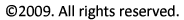Vectors

A vector is a quantity that has properties of magnitude (size) and direction. To represent this, we draw vectors as arrows, where the vector magnitude is indicated by the length of the arrow and the direction of the vector is indicated by the arrow orientation. Common vectors that occur in propulsion are forces (like thrust and drag), velocity, and acceleration.

Many times multiple vectors are involved in a problem and we need to find their "net" or "resultant" effect. To find the resultant vector we must use vector addition (summation). Vector addition is different from addition of two numbers because we must account for both the magnitude and direction of the vectors.

A common example is summation of forces acting on an object to find the resultant or "net" force on the object. This is illustrated in the figure below where two forces are pushing on a block. One force has a magnitude of 30 N in the x direction and the other force has a magnitude of 40 N in the y direction. Because these forces are in different directions, we cannot simply add their magnitudes to get the resultant force.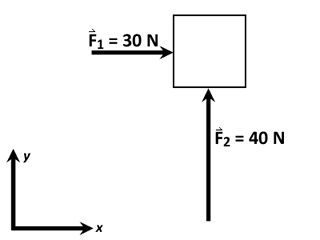Schematic of forces on a block

Instead, we can add these vectors graphically as shown in the figure below. Graphical vector addition proceeds by aligning the two vectors head to tail. Then the vector sum or resultant vector is the vector drawn from the tail of the first vector to the head of the last vector.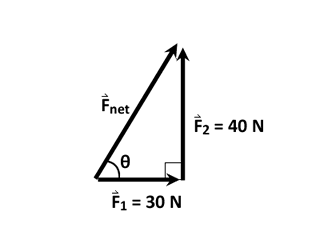Mathematically we can use the the Pythagorian theorem to find the magnitude of the resultant force. Likewise, we can use trigonometry to find the direction of the resultant force, expressed as the angle of the force, θ, relative to the horizontal. The appropriate calculations are given below.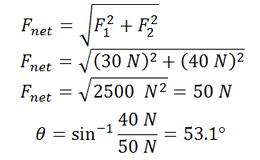Vector Notation: Sometimes it is inconvenient to represent vectors graphically as arrows. Instead, we often represent vectors symbolically using vector notation. One common method is to represent a vector as a combination of its components in the x, or "i", direction and the y, or "j", direction. For the case above, the net force fector is expressed as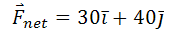where the i and j symbols serve to remind us of the direction associated with each component magnitude and the small arrow over Fnet indicates it is a vector.

Alternatively, we can simply indicate the magnitude and direction of the vector directly as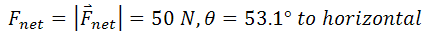Note that the vector magnitude can be represented either by placing vertical bars around a vector or by using the vector symbol in italics without an arrow over it.

Vector Decomposition

Vector decomposition is the deconstruction of a vector that does not lie solely in one direction into a multiple vectors in different directions. Basically, vector decomposition is vector addition in reverse. Usually we decompose vectors into component vectors that are orthogonal. This must be done in such a way that the component vectors sum to the original vector.

To illustrate, consider the velocity vector below, which is 10 m/s at 53.1° relative to the horizontal. We would like to decompose this vector into its horizontal and vertical components.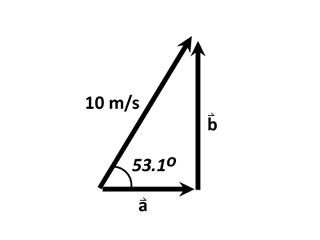Common Vector Decomposition Problem

Using basic trigonometry, we can directly calculate the magnitude of the component vectors in both the horizontal (i) and vertical (j) directions. Using the sine function, the magnitude of the vertical component can be determined as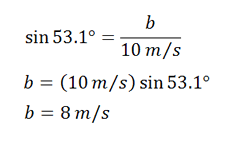Then, using the Pythagorian theorem it is possible to determine the remaining leg of the vector triangle as follows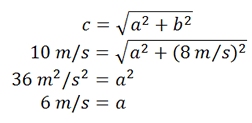Alternatively, trigonometry could be used again to determine the third leg of the triangle, namely,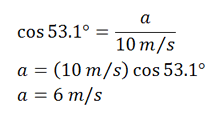Often it is convenient to decompose vectors into their components when only one of the components is important for a problem. Additionally, knowing the horizontal and vertical components of a vector allows us to represent the vector in vector notation. Using our results, the velocity vector considered above can be written in vector notation as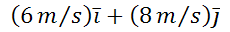Relative Motion and Vectors

Vectors are also useful in understanding the concept of relative motion. To illustrate how vectors are used in determining relative motion, we'll determine the relative wind velocity observed from a moving sailboat.

The sailboat shown below sails to the northeast at 20 knots while the wind blows from the east at a steady 10 knots. What is the direction and the magnitude of the wind velocity felt (observed) by the sailors aboard the sailboat?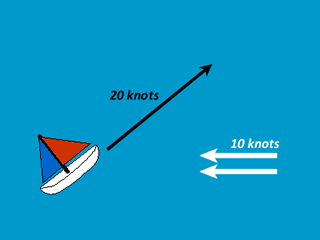Relative wind velocity illustration

To determine the wind velocity observed by the sailors, we must consider the problem from their point of view. Specifically, they see the ocean moving by them at 20 knots from the bow of the boat to the stern, meaning that they see the ocean moving by in the southwest direction at 20 knots. Adding the southwesterly motion observed by the sailors to the 10 knot westerly motion of the wind gives the wind velocity observed by the sailors. The graphical vector addition is illustrated in the figure below.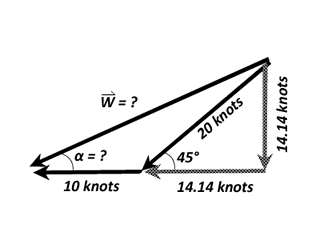Because the velocity of the sailboat is at a 45° angle, it will be easier to find the magnitude (W) and direction of the relative wind velocity if we first decompose the sailboat velocity vector into its horizontal and vertical components. The component vectors are shown in the figure above. Because the sail boat velocity is at a 45° angle, the magnitudes of its horizontal and vertical components are equal and easily determined from the Pythagorean theorem as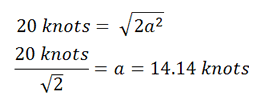After having decomposed the sailboat velocity into its components, it is apparent that the horizontal component of the relative wind velocity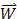is the sum of the two horizontal components (10 m/s and 14.1 m/s) while the vertical component ofis the same as the vertical component of the sailboat velocity. So, invector notation we have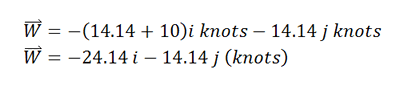Vector calculations

Notice that "−" signs are used to indicate that the vertical component points odwn and the horizontal components point to the left. Likewise, the magnitude and direction of W can be determined as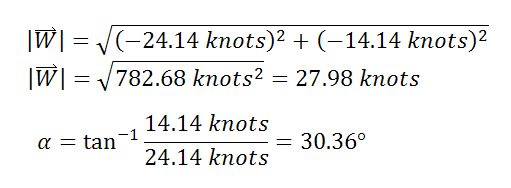Magnitude and direction calculations